• QualiFLY™
• World of Big Data
• Examination
• Get Started
×
Managing Risks Using Monte Carlo Simulations

# Insights

## Managing Risks Using Monte Carlo SimulationsA view of the business world before Data Science:

Uncertainties plagued decision-makers. CEOs, CFOs, investors, board members, and decision-makers projected their own experiences, incentives, and vision to arrive at decisions. These projections fell-far from the actual outcomes most of the time. Decision making was unanimously agreed upon as a risky and frustratingly organic process.

Enter Data Science:

Methods like Monte Carlo Simulations, regression and feature selection made risk-assessment more dependable and logical.

We will cover the above three in separate posts and limit this one to Monte Carlo Simulations.

The origin of Monte Carlo Simulations

Monte Carlo Simulations have their physical origin in the Monte Carlo of Monaco, known worldwide for its casinos, and their conceptual origin in mathematical and physics problems that couldn’t be solved with known approaches.

These simulations have found use in business, engineering, R&D, finance, trading, project management and insurance and their most common use has been in solving problems of optimization, finding draws from a probability distribution, and numerical integration.

Monte Carlo Simulations estimate the risk and uncertainty in forecasting models.

Random walk

Random walk serves as the conceptual foundation for Monte Carlo Simulations.

A random walk is a random path that a mathematical object takes in mathematical space. For example, on an integer line, a number from 0 can either go to +1 or -1 and the probability will be the same in both the cases.

Random walk, as the name suggests, points out to random outcomes of an event.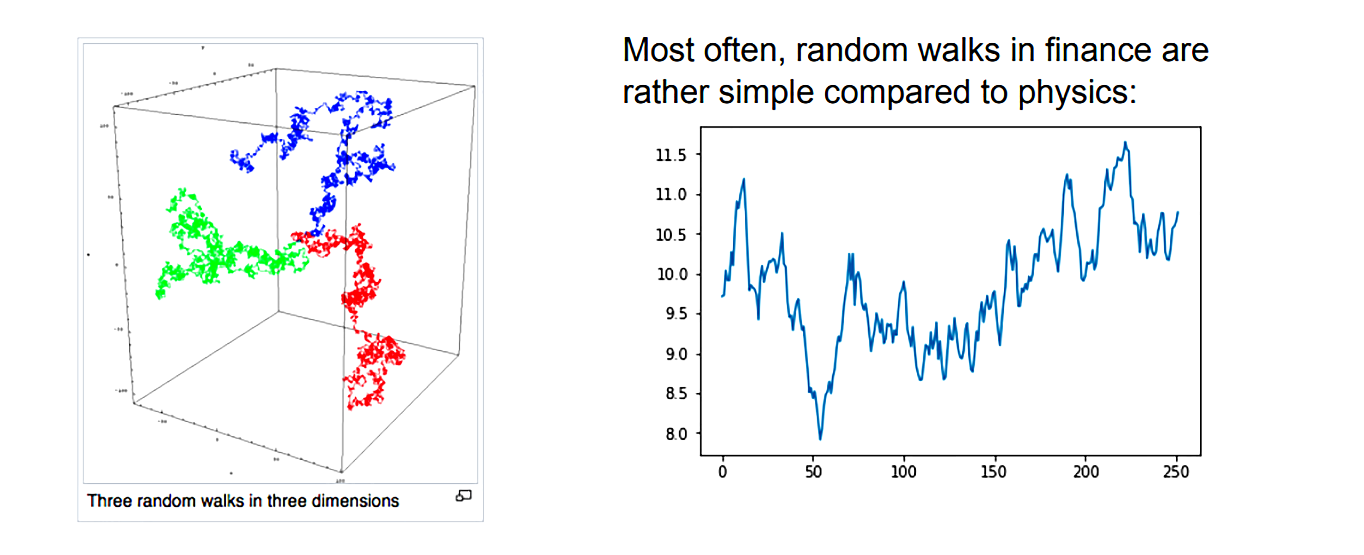Some common scenarios wherein random walk is used include identifying the path of a molecule as it moves in liquid or gas medium, knowing the path of a wild animal or knowing the price fluctuations in stock.

To generate a random walk in python, the following libraries are needed:

Matplotlib: To plot the curve. The code to install this library is

pip install matplotlib

Numpy: To work with array and matrices. The code to install NumPy is

pip install numpy

Random: To generate random points. A built-in library in python.

Monte Carlo Simulation

Data Science professionals use Monte Carlo Simulations to get insights into future events.

Take the example of a dice with 1 to 6 numbers. On each roll, the dice will show one of these numbers. When the dice is rolled thousands of times we will get its probability of rolling to a specific number with each succeeding roll. Monte Carlo Simulation helps us perform several such “what if” scenarios. The mathematical formula for calculation is:Drift is the direction in which the asset has been inclined to in the past.

Simulation for a Casino Bet

Let’s understand the application of Monte Carlo Simulations from the quintessential example of a casino:

Consider an imaginary roulette game with outcomes between 1 and 100. If the player rolls between 1-51, the house wins and if the player rolls between 52-100, the player wins.

Use these five steps to generate scenarios:

Step1: Import random and matplotlib libraries

Step 2: Create a dice simulator throwing values between 1-100 and considering 52-100 as ‘True’ and 1-51’ as ‘False.’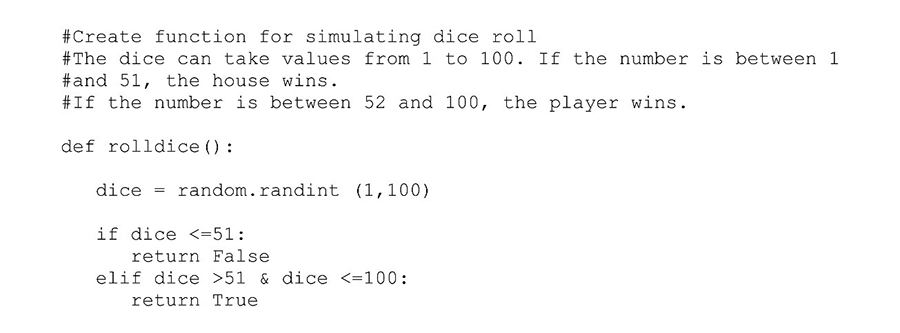Step 3: Create a function simulating the bets assuming the total funds to be USD 10,000 and wager amount USD 100.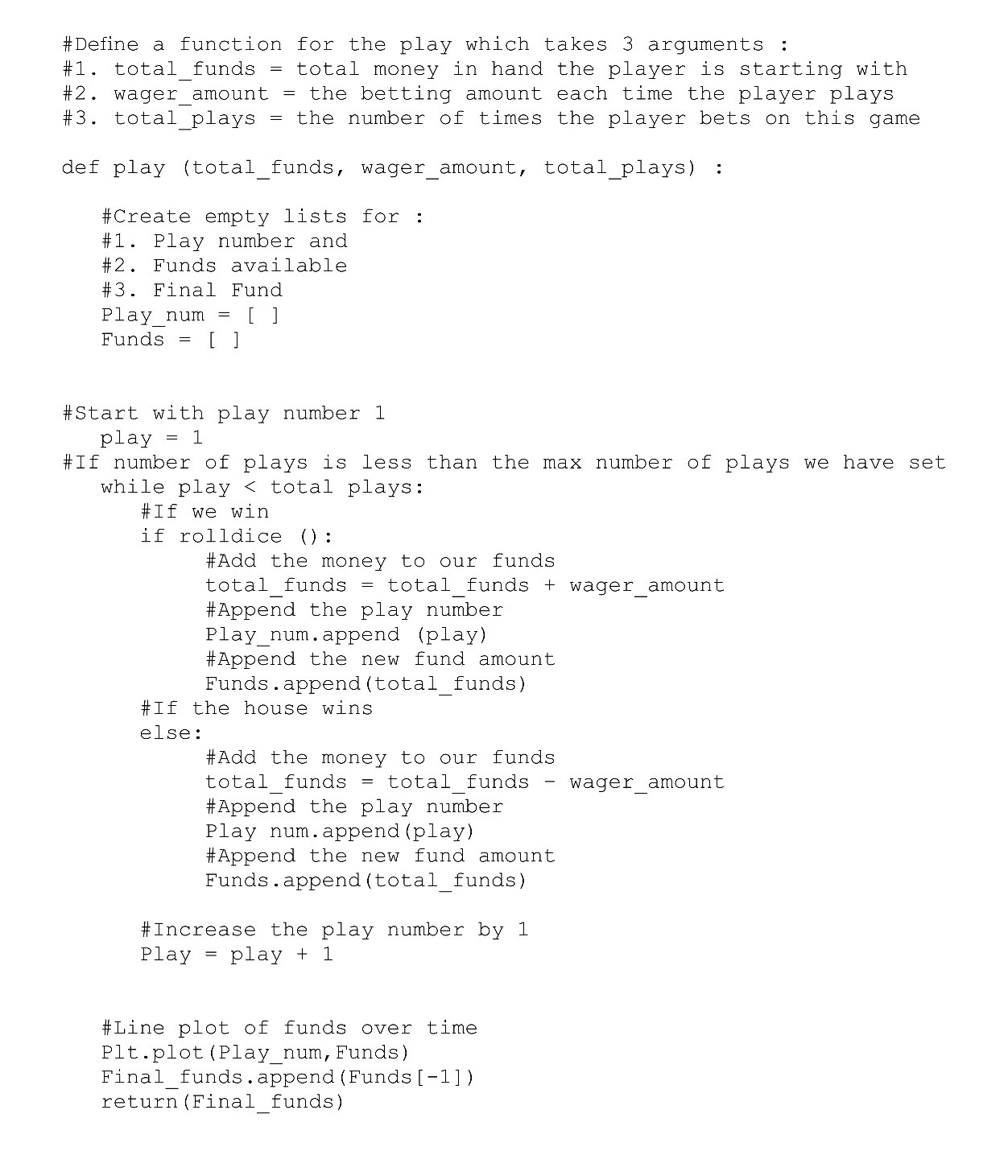Step 4: Run the loop for different scenarios.Step 5: Generate the scenarios: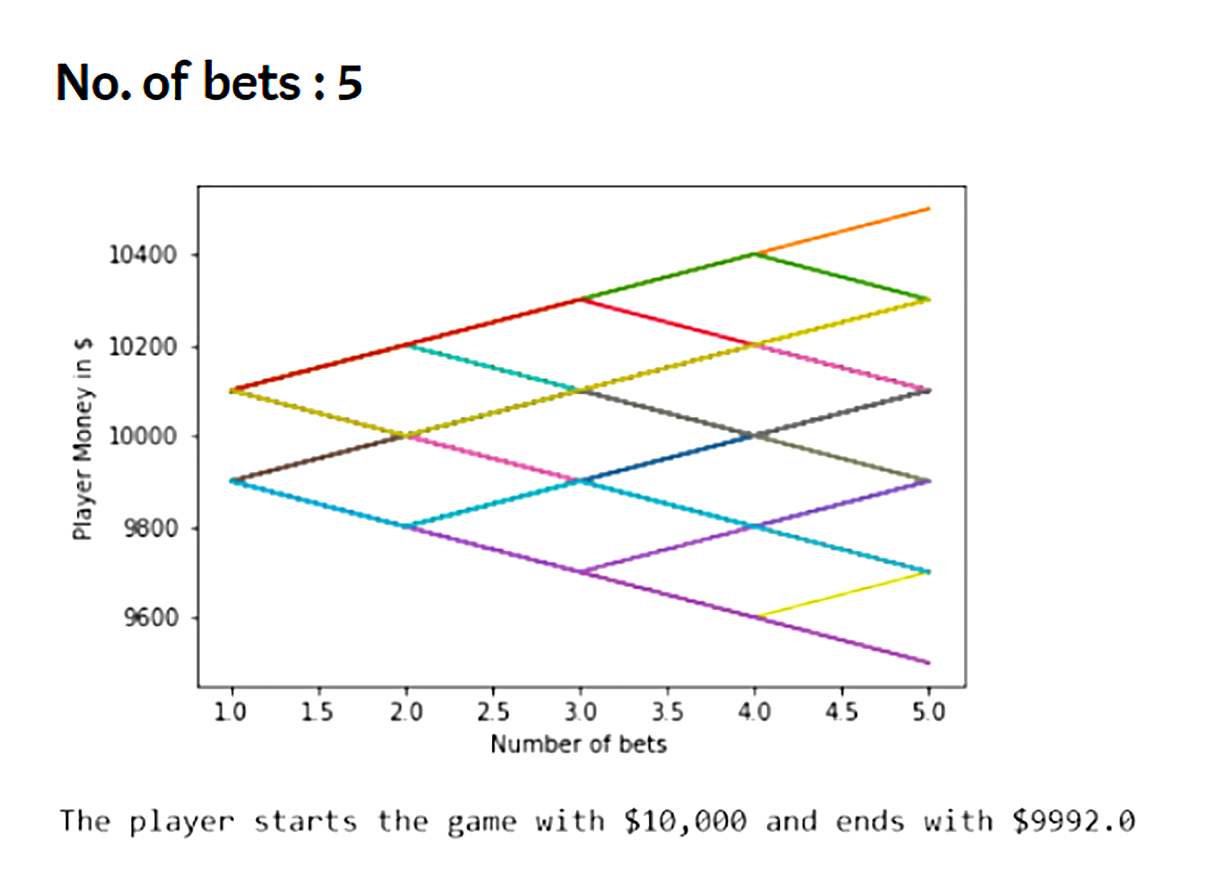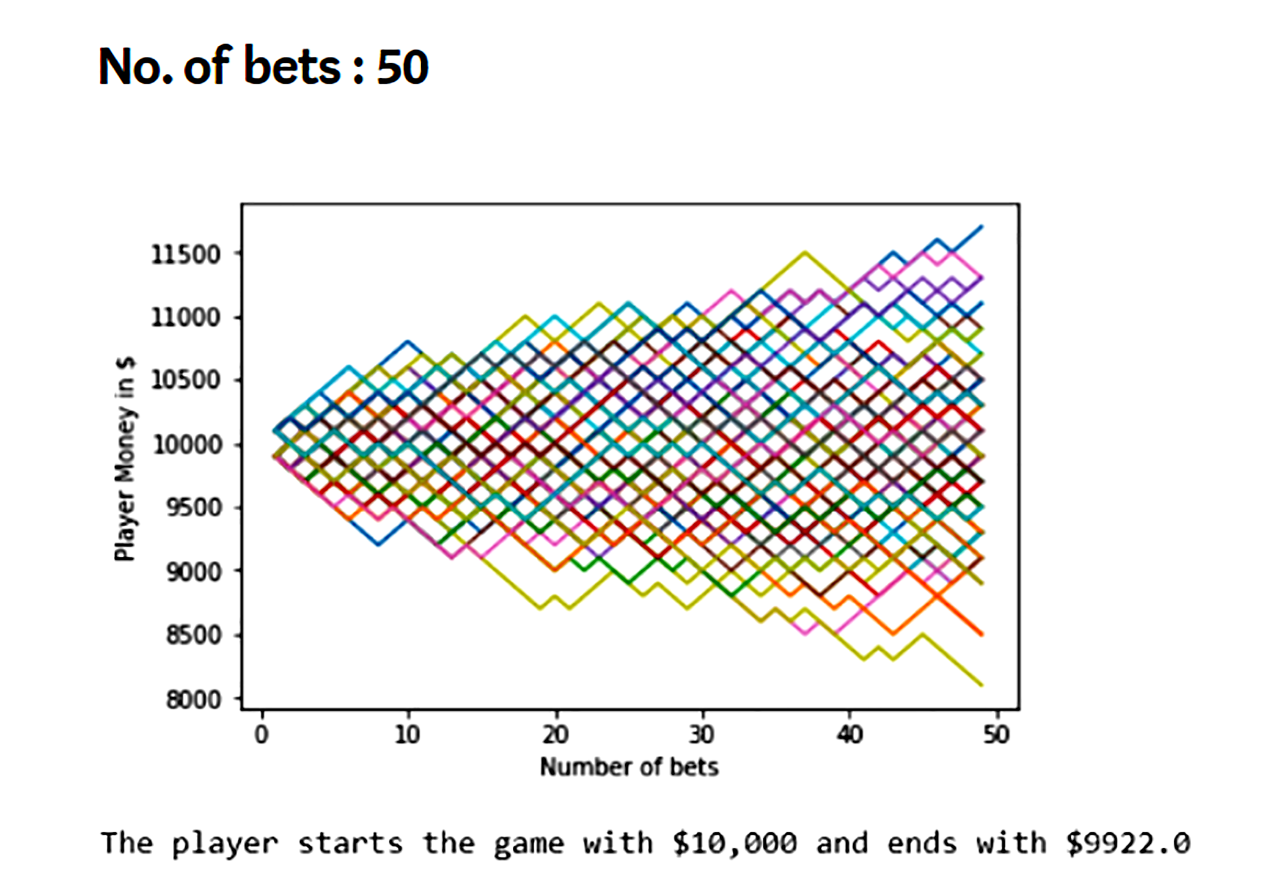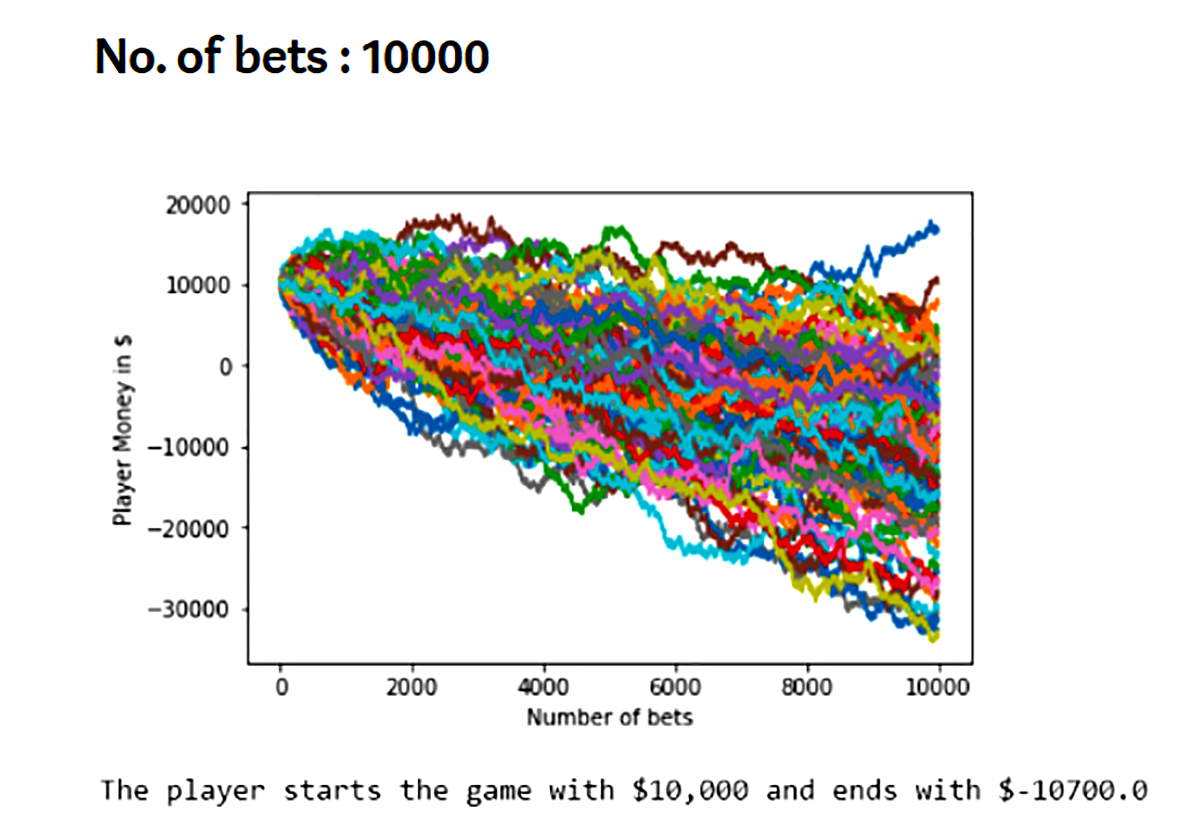Tip-Offs

The greatest advantage of the Monte Carlo method i.e. it takes into account multiple possible events sometimes results in its biggest disadvantage as it fails to predict extreme events.

While Monte Carlo Simulations can be performed to a limited extent in Microsoft excel, with python, R, and programming languages thousands of outcomes can be generated.

Output in Monte Carlo Simulations is as good as the inputs.

> <